# Symbol and Form

PATTERN, CYCLE, PROCESS, FUNCTION, MEANING

There is geometry in the humming of the strings
… there is music in the spacing of the spheres. -Pythagoras

## ______________________________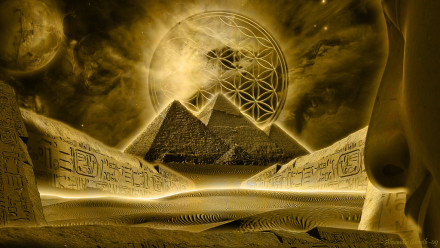This is an extensive section that is in the process of being built. Come back to see what gets uploaded, written and connected.

FORM

Form is integral to living systems, to life, to Nature-Cosmos. Form, also, is inextricably linked with its functionality, with the expression, the service or the role it exists to fulfill.

Form exhibits meaning.

Nothing in living systems – no entity, event, system, process or form – exists without meaning that not only serves its own function but also coherently, intelligently and cooperatively serves the Whole simultaneously.

SYMBOL

Symbols are material forms or formed acts which reflect and contain immaterial information. They connect to our right brain and Archetypal Mind.

_________________________________This image shows the geometrical form of “The Flower of Life” within which is the symbol of the Kabbalah Tree of Life.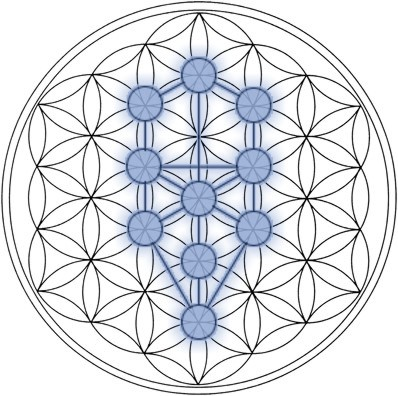The Flower of Life is named for a flower-like geometrical pattern that emerges from form that intersects in coherent relationships. It is a figure composed of multiple evenly-spaced, overlapping circles and it forms a symmetrical structure of a hexagon.

The Flower of Life symbol consists of seven or more overlapping circles, in which the center of each circle is on the circumference of up to six surrounding circles of the same diameter.

Above, we see the Kabbalah Tree of Life, (“Etz Hayim” / “Etz Chaim”) seamlessly embedding within “The Flower”. The Tree of Life symbol was created to reflect the 22 primeval vibrations that formed the Hebrew Alphabet. Thus, sound, form and meaning are all coordinated in one cohesive whole. This just refers to “The Tree” itself: the fact that it fits so perfectly within “The Flower” reinforces some fundamental principle and foundational meaning.

The symbol of The Flower of Life has been used and is evident throughout countless ancient cultures around the world.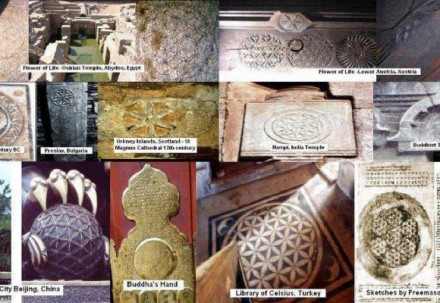Due to the diverse use throughout disparate cultures, there would be an apparent understanding of information contained in and transferred by these symbols. Take a look at the images below which follow a progression exemplifying the start of life. Could it be said that the foundations of life were understood? Further, why did so many ancient peoples find it necessary to reflect this understanding by embedding the symbology into significant cultural or architectural works?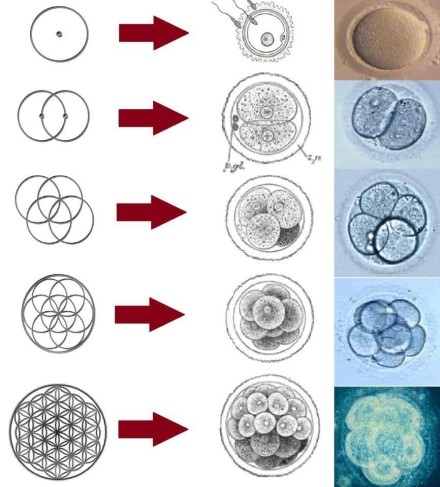Below is an image of a 64 Tetrahedron Grid, which reflects-becomes The Flower of Life. Physicist Nassim Haramein says of the Tetrahedron: “This is possibly the only possible genuine three-dimensional fractal geometry and it is unique in the sense that it naturally creates vector equilibrium at harmonic intervals – this is the geometry of space….The 64 tetrahedron matrix consists of terahedrons and octahedrons. A tetrahedron is the smallest possible geometric volume and constitutes a basic form with minimal energy consumption vs. optimal structural stability.”

This idea of minimal energy and optimal stability, although different, is reflective of, as theoretical biologist Brian Goodwin would say, “maximal individual freedom and maximal collective order”. Both exist; both reflect optimized function; both speak to the inherent efficacy and “both-and” coherence at the foundation of all living systems. Least effort and optimal functionality, individual-collective : this is the language of Nature-Cosmos, of life.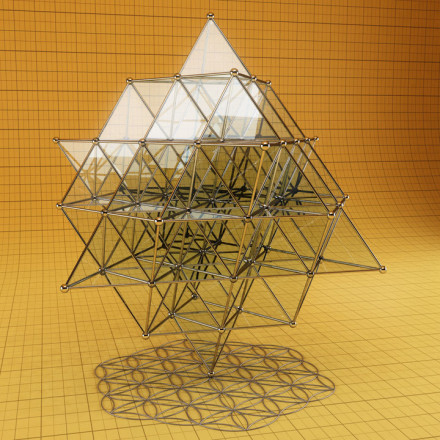Says Haramein: “Moving towards the infinitely large or the infinitesimally small, the underlying matrix remains in structural equilibrium within its own scale. The picture below shows a tetrahedron and a polarized star tetrahedron, then we see the first three iterations of the fractal; its minimum size in the form of the 64 tetrahedron crystal, then 512 tetrahedron, then 1026 tetrahedron.”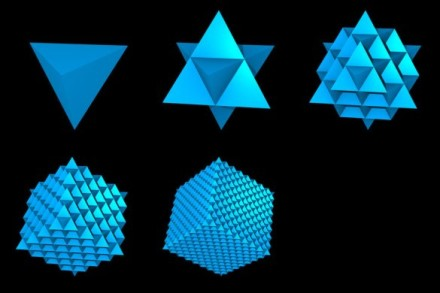_____________________________________________

All material form is reflective of immaterial principles and all immaterial principles are coherent and reflective of a blueprint, of an evolutionary progression. Below is an example.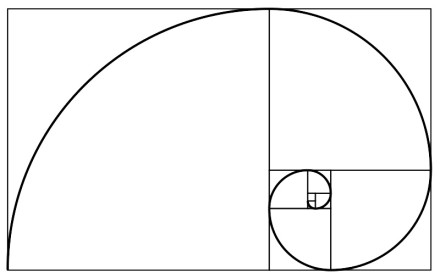The above graphic is one you’ll recognize, the Fibonacci Spiral. This form is existent and evident throughout Nature-Cosmos.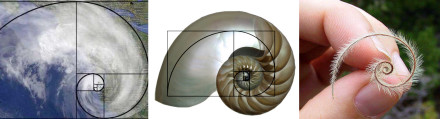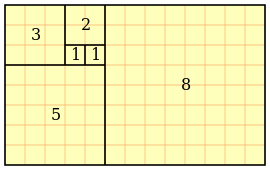Using the integer sequence –   0, 1, 1, 2, 3, 5, 8, 13, 21, 34, 55, 89, 144 and so on – the Fibonacci Spiral is created by drawing circular arcs connecting the opposite corners of squares in the Fibonacci tiling.

The “tiling” consists of squares where the side lengths are successive Fibonacci numbers reflecting the Fibonacci Sequence.

This sequence is based on numerical additions and the subsequent coherent pattern that emerges. Through numbers, we see form, we see nature’s expansion-tendency, and we see intelligent meaning embedded in symbol and form throughout Nature-Cosmos.

1+1=2, 2+1=3, 3+2=5, 5+3=8, 8+5=13, etcetera.

The Fibonacci sequence is named after Fibonacci (also known as Leonardo of Pisa). His 1202 book Liber Abaci introduced the sequence to Western European mathematics, although the sequence had been described earlier in Indian mathematics.

– More on the Indian origins will be posted soon. –

_____________________________________________

NUMBERS

“If you only knew the magnificence of the 3, 6 and 9, then you would have a key to the universe.” – Nikola Tesla

Aristotle, in his work  Metaphysica, says of the Pythagorean understanding of numbers:

“The (Pythagoreans were) … the first to take up mathematics … (and) thought its principles were the principles of all things. Since, of these principles, numbers … are the first, … in numbers they seemed to see many resemblances to things that exist … more than [just] air, fire and earth and water, (but things such as) justice, soul, reason, opportunity …”

According to the Pythagorean understanding of numbers (the information below from Dartmouth.edu), the functionality and meanings were:

1   Monad. Point. The source of all numbers. Good, desirable, essential, indivisible.

2   Dyad. Line. Diversity, a loss of unity, the number of excess and defect. The first feminine number. Duality.

3   Triad. Plane. By virtue of the triad, unity and diversity of which it is composed are restored to harmony. The first odd, masculine number.

4   Tetrad. Solid. The first feminine square. Justice, steadfast and square. The number of the square, the elements, the seasons, ages of man, lunar phases, virtues.

5   Pentad. The masculine marriage number, uniting the first female number and the first male number by addition.

• The number of fingers or toes on each limb.
• The number of regular solids or polyhedra.

Incorruptible: Multiples of 5 end in 5.

6   The first feminine marriage number, uniting 2 and 3 by multiplication. The first perfect number (One equal to the sum of its aliquot parts, IE, exact divisors or factors, except itself. Thus, (1 + 2 + 3 = 6). The area of a 3-4-5 triangle

7   Heptad. The maiden goddess Athene, the virgin number, because 7 alone has neither factors or product. Also, a circle cannot be divided into seven parts by any known construction).

8   The first cube.

9   The first masculine square. Incorruptible – however often multiplied, reproduces itself.

10   Decad. Number of fingers or toes. Contains all the numbers, because after 10 the numbers merely repeat themselves. The sum of the archetypal numbers (1 + 2 + 3 + 4 = 10)

27   The first masculine cube.

28   Astrologically significant as the lunar cycle. It’s the second perfect number (1 + 2 + 4 + 7 + 14 = 28). It’s also the sum of the first 7 numbers (1 + 2 + 3 + 4 + 5 + 6 + 7 = 28)!

35   Sum of the first feminine and masculine cubes (8+27)

36   Product of the first square numbers (4 x 9). Sum of the first three cubes (1 + 8 + 27). Sum of the first 8 numbers (1 + 2 + 3 + 4 + 5 + 6 + 7 + 8)

FIGURED NUMBERS

The Pythagoreans represented numbers by patterns of dots, probably a result of arranging pebbles into patterns. The resulting figures have given us the present word figures.

Thus 9 pebbles can be arranged into 3 rows with 3 pebbles per row, forming a square.

Similarly, 10 pebbles can be arranged into four rows, containing 1, 2, 3, and 4 pebbles per row, forming a triangle.

From these they derived relationships between numbers. For example, noting that a square number can be subdivided by a diagonal line into two triangular numbers, we can say that a square number is always the sum of two triangular numbers.

Thus the square number 25 is the sum of the triangular number 10 and the triangular number 15.

SACRED TETRACYTS

One particular triangular number that they especially liked was the number ten. It was called a Tetractys, meaning a set of four things, a word attributed to the Greek Mathematician and astronomer Theon (c. 100 CE). The Pythagoreans identified ten such sets.

TEN SETS OF FOUR THINGS

 Numbers 1 2 3 4 Magnitudes point line surface solid Elements fire air water earth Figures pyramid octahedron icosahedron cube Living Things seed growth in length in breadth in thickness Societies man village city nation Faculties reason knowledge opinion sensation Seasons spring summer autumn winter Ages of a Person infancy youth adulthood old age Parts of living things body three parts of the soul

_____________________________________________

The video here gives a glimpse of the numerical imprints which underlie living systems. These act to “inform” life: in-Form.

_____________________________________________

As the deeper implication of numbers comes more into focus, a process (mental-intuitive) begins that allows the conversation to unfold further. A friend of mine, a uniquely innovative mathematical brain, started diving into the idea of numbers as symbols and forms with distinct function and fundamental meaning. The start of a dialogue and journey. See his real-time exploration on video here.